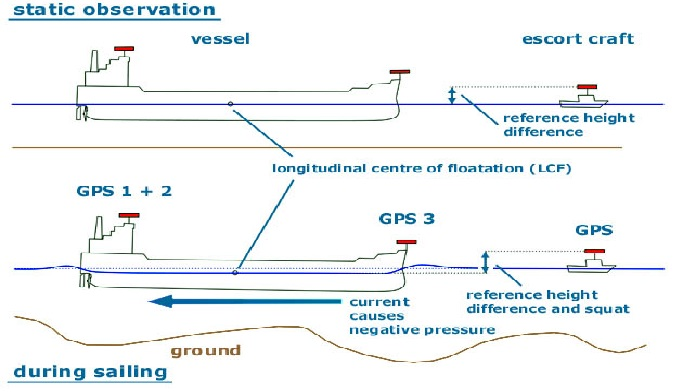# What is Squat?

The water displaced by a moving hull is not easily replaced when the hull moves through shallow water hence the bow wave and the stern wave of the vessel increase in height and the trough which normally exist at the quarters deepens and the after part of the vessel is drawn downwards towards the bottom as a result Under Keel Clearence decreases. This is called squat.

Factors governing squat:

• Squat varies on the following factors:
• Ship’s speed: Squat is directly proportional to the square of speed.
• Block co-efficient: Squat directly varies with CB.
• Blockage factor (S): It is the ratio between cross section of the vessel and cross section of the canal or river.

So, in confined water, squat is more than in open water.

Squat may be calculated by the following simplified formulae:

• Squat = (CB X V2 ) / 100 (In open waters)
• Squat = 2 X (CB X V2 ) / 100 (In confined waters)

Precaution

• Squat may cause grounding in spite of enough UKC.
• Squat to be calculated beforehand.
• Speed to be reduced to reduce squat.
• While determining UKC, squat for the speed to be taken into consideration.